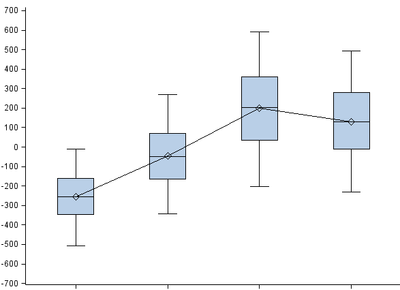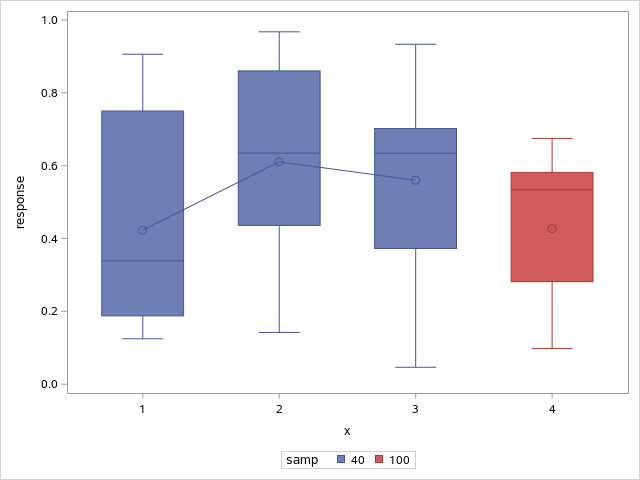## Boxplot comparing different samples- only connecting some groups

The first three boxes are groups from the same sample while the last box is from a different sample entirely. Is it possible to keep this graph the same but have the line not connect to the last box? I used proc sgplot to generate the graph connecting on mean. Any help is much appreciated! Thank you in advance!2 REPLIES 2

## Re: Boxplot comparing different samples- only connecting some groups

As a minimum the code used to create that graph would be helpful.

Since there are very few plots that can mix with the VBOX you may want to turn of the connection in VBOX and create an annotate data set to connect the 3 desired mean values for the categories.

Another option might be to have a different analysis variable for the "other" data and have two vbox statements. Make sure that the two analysis variables are only assigned for the appropriate category values.

An example with made up data to demonstrate principal:

```data example;
do group= 1 to 3;
do i= 1 to 20;
y1 = rand('uniform');
output;
end;
end;
/* clear for example data the y1*/
y1=.;
group=4;
do i=1 to 20;
y2= rand('uniform');
output;
end;
run;

proc sgplot data=example;
vbox y1 / connect=mean category=group ;
vbox y2 / category=group;
run;```

Note that because the categories have missing values for the analysis variables you will get notes in the log about incomplete or invalid data and "some boxes will not be drawn".

You will likely want to suppress the legend, or modify it. By default the two sets of boxes are likely to also be in different colors.DanH_sas
SAS Super FREQ

## Re: Boxplot comparing different samples- only connecting some groups

I think the best option to display what you describe is to use a boxplot grouped by sample. Here is a little program to simulate the idea:``````data sample;
do x=1 to 4;
if x < 4 then samp=40;
else samp=100;
do t=1 to 10;
response=ranuni(123);
output;
end;
end;
run;

proc sgplot data=sample;
vbox response / category=x group=samp groupdisplay=overlay connect=mean;
run;
``````

If you don't want the color change, the other option is to do as @ballardw suggested, and that was pre-compute the mean and overlay an annotated line or a SERIES plot.

Hope this helps!

Dan

Discussion stats
• 2 replies
• 214 views
• 0 likes
• 3 in conversation